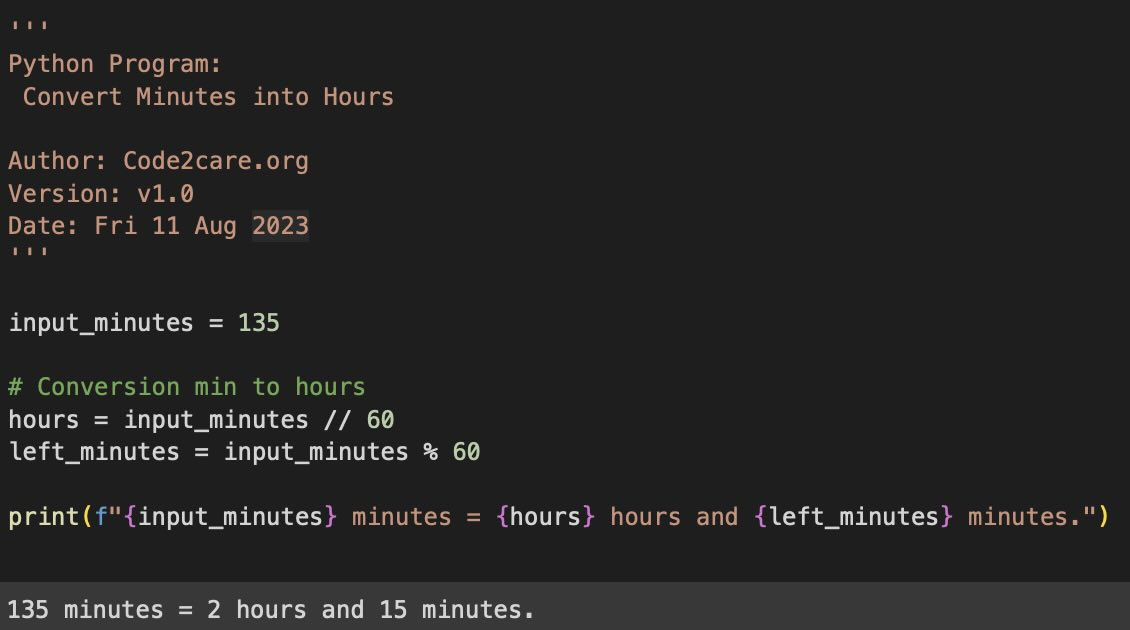# Python: Convert Minutes to Hours

If you want to convert time from minutes to hours using Python code then you need to apply the below pseudo-code,

## Pseudo Logic - Convert Minutes into Hours

1. Calculate the hours by integer division

``Logic: hours = minutes // 60``
2. Next, calculate remaining minute using Modulus operator

``Logic: remaining_minutes = minutes % 60``

## Example 1: Using Hardcoded minutes

``````'''
Python Program:
Convert Minutes into Hours

Author: Code2care.org
Version: v1.0
Date: Fri 11 Aug 2023
'''

input_minutes = 115

# Conversion min to hours
hours = input_minutes // 60
left_minutes = input_minutes % 60

print(f"{input_minutes} minutes = {hours} hours and {left_minutes} minutes.")
``````
Output:
``````115 minutes = 1 hours and 55 minutes.

75 minutes = 1 hours and 15 minutes.

135 minutes = 2 hours and 15 minutes.``````## Example 2: User Inputs the Minutes

``````'''
Python Program:
Convert Minutes into Hours
from user input

Author: Code2care.org
Version: v1.0
Date: Fri 11 Aug 2023
'''

while True:
user_input = input("Enter the time in minutes ('e' to exit): ")

if user_input.lower() == 'e':
print("Program Exited.")
break

try:
input_minutes = int(user_input)
hours = input_minutes // 60
left_minutes = input_minutes % 60
print(f"{input_minutes} minutes = {hours} hours and {left_minutes} minutes.\n")
except ValueError:
print("Invalid input.\n")``````
Output:
``````Enter the time in minutes ('e' to exit): 99
98 minutes = 1 hours and 39 minutes.

Enter the time in minutes ('e' to exit): 110
110 minutes = 1 hours and 50 minutes.

Enter the time in minutes ('e' to exit): 245
245 minutes = 4 hours and 5 minutes.

Enter the time in minutes ('e' to exit): e
Exiting the program.``````
-

### Facing issues? Have Questions? Post them here! I am happy to answer!

Author Info:

Rakesh is a seasoned developer with over 10 years of experience in web and app development, and a deep knowledge of operating systems. Author of insightful How-To articles for Code2care.